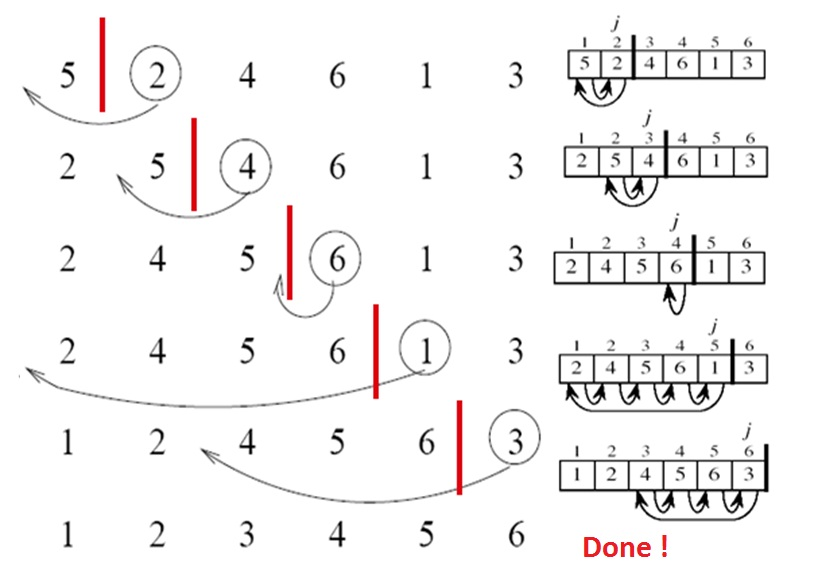# Algorithm Basics

suggest change

Insertion sort is a very simple, stable, in-place sorting algorithm. It performs well on small sequences but it is much less efficient on large lists. At every step, the algorithms considers the i-th element of the given sequence, moving it to the left until it is in the correct position.

Graphical IllustrationPseudocode

``````for j = 1 to length(A)
n = A[j]
i = j - 1
while j > 0 and A[i] > n
A[i + 1] = A[i]
i = i - 1
A[i + 1] = n``````

Example

Consider the following list of integers:

``[5, 2, 4, 6, 1, 3]``

The algorithm will perform the following steps:

1. [5, 2, 4, 6, 1, 3]
2. [2, 5, 4, 6, 1, 3]
3. [2, 4, 5, 6, 1, 3]
4. [2, 4, 5, 6, 1, 3]
5. [1, 2, 4, 5, 6, 3]
6. [1, 2, 3, 4, 5, 6]